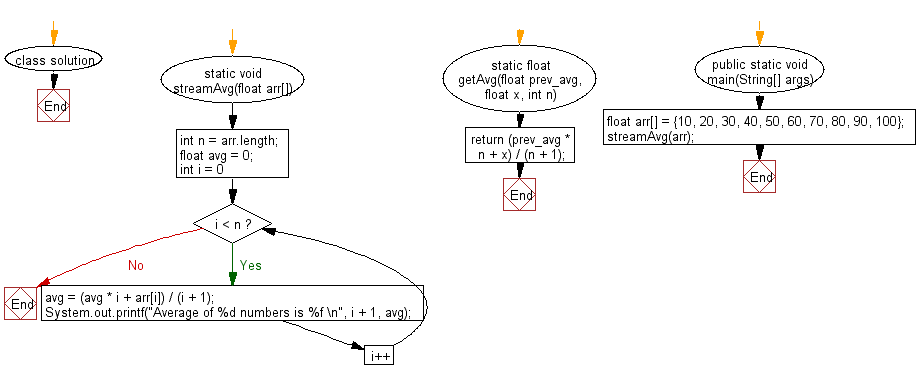﻿ Java Math Exercises: Calculate and print average of the stream of given numbers - w3resource# Java Math Exercises: Calculate and print average of the stream of given numbers

## Java Math Exercises: Exercise-17 with Solution

Write a Java program to calculate and print average (or mean) of the stream of given numbers.

Sample Solution:

Java Code:

``````import java.util.*;
class solution {
// Prints average of a stream of numbers
static void streamAvg(float arr[])
{
int n = arr.length;
float avg = 0;
for (int i = 0; i < n; i++)
{
//avg = getAvg(avg, arr[i], i);
avg = (avg * i + arr[i]) / (i + 1);
System.out.printf("Average of %d numbers is %f \n",
i + 1, avg);
}
return;
}

// Calculate the new average
static float getAvg(float prev_avg, float x, int n)
{
return (prev_avg * n + x) / (n + 1);
}

public static void main(String[] args)
{
float arr[] = {10, 20, 30, 40, 50, 60, 70, 80, 90, 100};
streamAvg(arr);
}
}
``````

Sample Output:

``` Average of 1 numbers is 10.000000
Average of 2 numbers is 15.000000
Average of 3 numbers is 20.000000
Average of 4 numbers is 25.000000
Average of 5 numbers is 30.000000
Average of 6 numbers is 35.000000
Average of 7 numbers is 40.000000
Average of 8 numbers is 45.000000
Average of 9 numbers is 50.000000
Average of 10 numbers is 55.000000
```

Flowchart:Java Code Editor:

What is the difficulty level of this exercise?

﻿

## Java: Tips of the Day

Parsing dates:

```import java.io.*;
import java.util.*;
import java.text.*;

String s = "2001/09/23 14:39";

SimpleDateFormat formatter = new SimpleDateFormat ("yyyy/MM/dd H:mm");
Date d = formatter.parse(s, new ParsePosition(0));
```